Courses

# Match the following of Electrochemistry, Past year Questions JEE Advance, Class 12, Chemistry JEE Notes | EduRev

## Class 12 Chemistry 35 Years JEE Mains &Advance Past yr Paper

Created by: Abhishek Kapoor

## JEE : Match the following of Electrochemistry, Past year Questions JEE Advance, Class 12, Chemistry JEE Notes | EduRev

The document Match the following of Electrochemistry, Past year Questions JEE Advance, Class 12, Chemistry JEE Notes | EduRev is a part of the JEE Course Class 12 Chemistry 35 Years JEE Mains &Advance Past yr Paper.
All you need of JEE at this link: JEE

Direction : question contains statements given in two columns, which have to be matched. The statements in Column-I are labelled A, B, C and D, while the statements in Column-II are labelled p, q, r, s and t.

Q.

Match the reactions in Columns I with nature of the reactions/type of the products in Column II. Indicate your answer by darkening the appropriate bubbles of the 4 × 4 matrix given in the ORS.

 Column I Column II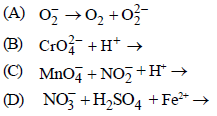(p) redox reaction (q) one of the products has trigonal planar structure (r) dimeric bridged tetrahedral metal ion (s) disproportionation

Ans:  A - p, s); (B - r); (C - p, q); (D - p).

Solution :  A→ p, s; The reaction is redox reaction because the O.N. of O in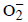is – 0.5 and that in O2 is zero. In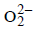is –1.0. It
involves reduction oxidation reaction. Since here a part of molecule is oxidised and a part is reduced so it is disproportionation.
B → r; The structure of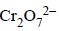is given below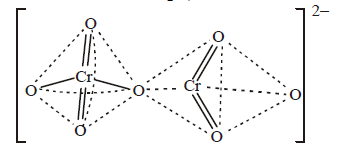[NOTE : In any solution dichromate ions and chromate ions exist in equilibrium. In alkali solution, dichromate ions are converted into chromate ions and on acidification chromate ions are converted back into dichromate ion.]
C → p, q; The reaction is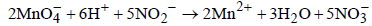In involves change in O.N of Mn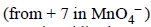to
+ 2(in Mn2+), So Mn is reduced and NO2–  is oxidised to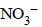it is a redox reaction. The structure ofit is a redox reaction.
– (one of the products is trigonal planar)
D → p, It is a redox reaction

Direction : questions have matching lists. The codes for the lists have choices (a), (b), (c) and (d) out of which ONLY ONE is correct.

Q.

An aqueous solution of X is added slowly to an aqueous solution of Y as shown in List I. The variation in conductivity of these reactions is given in List II. Match list I with List II and select the correct answer using the code given below the lists :                         (JEE Adv. 2013)

 List I List II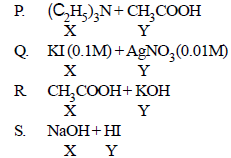1. Conductivity decreases and then increases2. Conductivity decreases and then does not change much3. Conductivity increases and then does not change much4. Conductivity does not change much and then increases

Codes: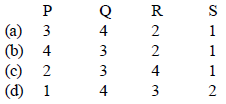Ans: a

Solution :  (a)
(p)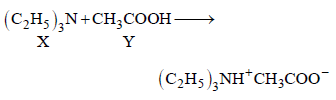Initially conductivity increases because on neutralisation ions are created. After that it becomes practically constant because X alone can
not form ions.

Q.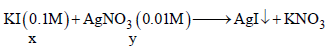Number of ions in the solution remains constant as only AgNO3 precipitated as AgI. Thereafter conductance increases due to increase in number
of ions.

(R) Initially conductance decreases due to the decrease in the number of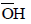ions as OH is getting replaced by CH3COO which has poorer conductivity
thereafter it slowly increases due to the increase in number of H+ ions.

(S) Initially it decreases due to decrease in H+ ions and then increases due to the increase in OH ions.

Q.

The standard reduction potential data at 25°C is given below : (JEE Adv. 2013)
E°(Fe3+, Fe2+) = + 0.77 V; E°(Fe2+, Fe) = – 0.44 V; E°(Cu2+, Cu) = + 0.34 V; E°(Cu+, Cu) = + 0.52 V
E°[O2(g) + 4H+ + 4e → 2H2O] = + 1.23 V; E°[O2(g) + 2H2O + 4e → 4OH] = + 0.40 V
E°(Cr3+, Cr) = – 0.74 V; E°(Cr2+, Cr) = – 0.91 V

Match E° of the redox pair in List I with the values given in List II and select the correct answer using the code given below the lists :

 List I List II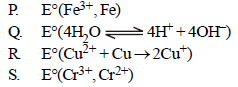1. – 0.18 V2. – 0.4 V3. – 0.04 V4. – 0.83 V

Codes :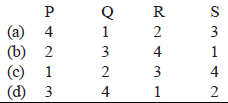Ans: d

Solution :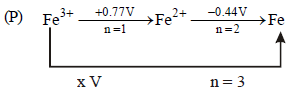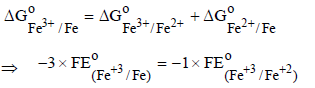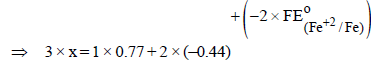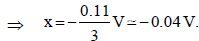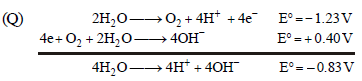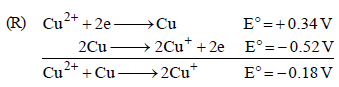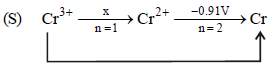– 0.74V, n = 3
x × 1 + 2 × (– 0.91) = 3 × (– 0.74)
x – 1.82 = – 2.22 ⇒ x = – 0.4V

Offer running on EduRev: Apply code STAYHOME200 to get INR 200 off on our premium plan EduRev Infinity!

69 docs|36 tests

,

,

,

,

,

,

,

,

,

,

,

,

,

,

,

,

,

,

,

,

,

,

,

,

,

,

,

,

,

,

;